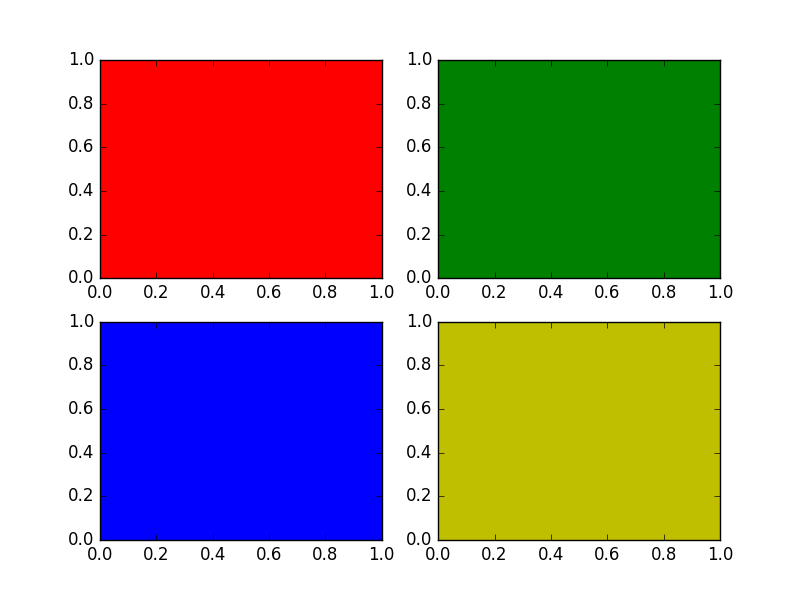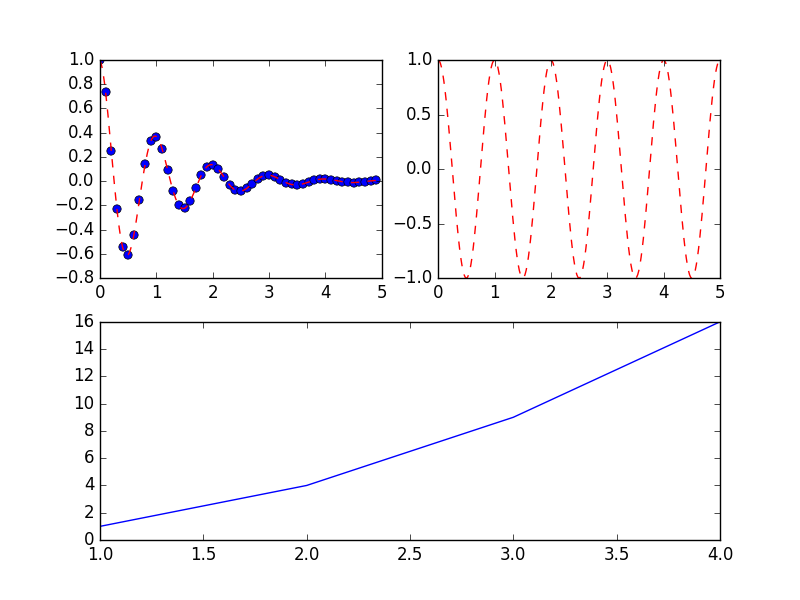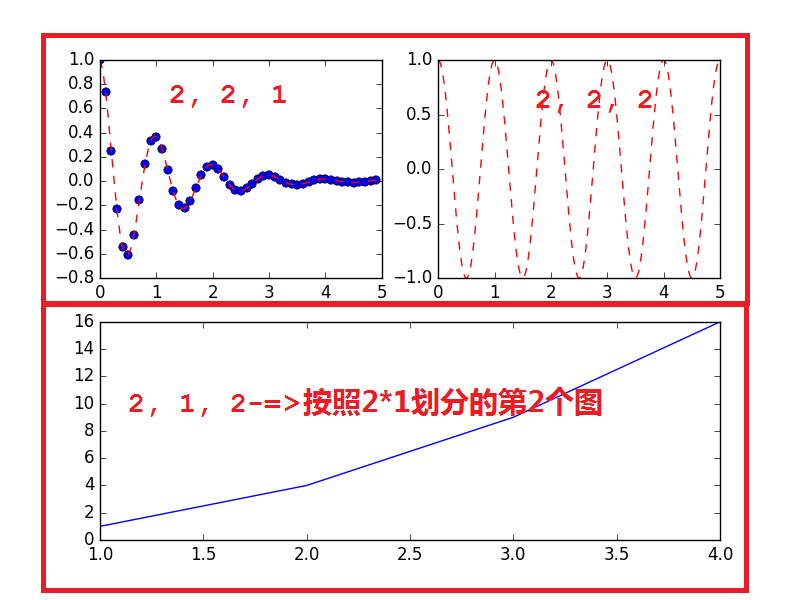# 1 问题描述

matploglib 能够绘制出精美的图表, 有些时候, 我们希望把一组图放在一起进行比较, 有没有什么好的方法呢?

matplotlib 中提供的 subplot 可以很好的解决这个问题

# 2 subplot函数介绍

matplotlib下, 一个 Figure 对象可以包含多个子图(Axes), 可以使用 subplot() 快速绘制, 其调用形式如下 :

subplot(numRows, numCols, plotNum)
• 图表的整个绘图区域被分成 numRows 行和 numCols

• 然后按照从左到右，从上到下的顺序对每个子区域进行编号，左上的子区域的编号为1

• plotNum 参数指定创建的 Axes 对象所在的区域

(1, 1), (1, 2), (1, 3)
(2, 1), (2, 2), (2, 3)

• 如果 numRows, numColsplotNum 这三个数都小于 10 的话, 可以把它们缩写为一个整数, 例如 subplot(323)subplot(3,2,3) 是相同的.

• subplotplotNum 指定的区域中创建一个轴对象. 如果新创建的轴和之前创建的轴重叠的话，之前的轴将被删除.

# 3 示例程序

## 3.1 规则划分成3*3的

#!/usr/bin/env python
#!encoding=utf-8

import matplotlib
import matplotlib.pyplot as plt

if __name__ == '__main__':
for i,color in enumerate("rgby"):
plt.subplot(221+i, axisbg=color)

plt.show()## 3.2 不规则划分#!/usr/bin/env python
#!encoding=utf-8

import matplotlib.pyplot as plt
import numpy as np

def f(t):
return np.exp(-t) * np.cos(2 * np.pi * t)

if __name__ == '__main__' :
t1 = np.arange(0, 5, 0.1)
t2 = np.arange(0, 5, 0.02)

plt.figure(12)
plt.subplot(221)
plt.plot(t1, f(t1), 'bo', t2, f(t2), 'r--')

plt.subplot(222)
plt.plot(t2, np.cos(2 * np.pi * t2), 'r--')

plt.subplot(212)
plt.plot([1, 2, 3, 4], [1, 4, 9, 16])

plt.show()

04-193万+

#### subplot和subplots绘制子图

03-122万+

#### python中subplot的用法08-175522

#### Python Matplotlib 关于subplot的使用

12-177458

#### 使用matplotlib:subplot绘制多个子图

07-02956

#### python使用matplotlib.subplot plt绘制多个子图

10-117万+

#### matplotlib绘制多个子图——subplot

01-161万+

#### matplotlib绘制多个子图——subplot的用法详解

06-271004

#### 【Matplotlib】详解图像各个部分

04-011万+

#### Python数据可视化matplotlib（二）—— 子图功能

03-181万+

#### matplotlib subplot函数介绍

07-29326

#### Subplot 多合一显示

08-233780

#### 九、matplotlib系列---pyplot极坐标图的绘制

12-024万+

01-162万+

05-272万+

#### matplotlib中使用subplots创建窗口如何设置尺寸大小

10-042527

#### Python--subplot 子图绘制©️2020 CSDN 皮肤主题: 大白 设计师: CSDN官方博客点击重新获取扫码支付1.余额是钱包充值的虚拟货币，按照1:1的比例进行支付金额的抵扣。
2.余额无法直接购买下载，可以购买VIP、C币套餐、付费专栏及课程。余额充值